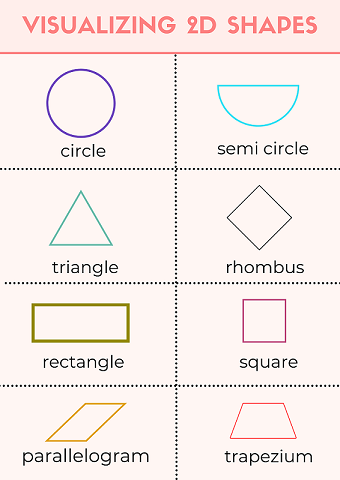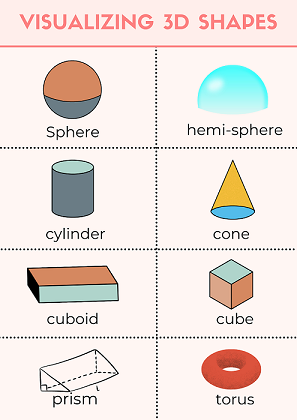Courses
Courses for Kids
Free study material
Free LIVE classes
More

# 2D and 3D Shapes: Types, Examples and Differences## What are 2-Dimensional and 3-Dimensional Shapes?

Everything we observe in the world has a shape. In the objects we see around us, we can find different basic shapes such as the two-dimensional square, rectangle, and oval, as well as the three-dimensional rectangular prism, cylinder, and sphere. Credit cards, notes and coins, finger rings, photo frames, dart boards, houses, windows, magician's wands, high buildings, flower pots, toys, and balloons are all examples of geometric shapes.

The number of sides or corners of a shape changes from one to another. A side, or vertex, is a straight line that forms part of a shape, and a corner, or vertex, is the point where two sides meet. You're already familiar with the most common shapes, and may not be able to explain the differences between a square and a cube, or a circle and a sphere. In this article you'll start analysing and comparing 2D and 3D shapes, explaining their similarities, differences, and properties.

## What are 2D shapes?

2D stands for two-dimensional in 2D shapes. Shapes with two dimensions, such as width and height, are known as 2D shapes. A rectangle or a circle are examples of 2D shapes because they don’t have depth. Basically 2D objects are flat and can't be physically held. We usually refer to dimensions as measurements in a specific direction. Length, width or breadth, depth, and height are examples of dimensions.

## Examples of 2D Shapes:

Circle

• There is just one bent side of a circle.

• A semi-circle has two sides, one curved and one straight.

• The full arc of the semi-circle measures 180 degrees.

Triangle

• An equilateral triangle is a triangle with each angle measuring 60°.

• Any triangle with one right angle is a right-angled triangle.

• An irregular triangle is a scalene triangle. All of the sides and angles are unique.

• Two sides and two angles of an isosceles triangle are the same.

• A square is a regular quadrilateral with 90o angles on all sides.

• The diagonals of a kite intersect at right angles and have two sets of equal length sides.

• A rectangle is made up of two sets of parallel straight lines, each with a 90° angle.

• A rhombus has equal sides and opposite equal angles, as well as two sets of parallel lines.

• One pair of parallel lines makes up a trapezium.

• Two pairs of parallel lines with opposite equal angles make up a parallelogram.

###Image Shows the Illustration of 2D Shapes

## 3D Shapes

3D means it has three dimensions. So , it isn't flat like a 2D shape. The dimensions of 3D shapes include length, breadth, and depth. Examples of 3D shapes include spheres, cuboids, cubes, square-based pyramids, cylinders, and cones.Image Shows the Illustration of 3D Shapes

## Properties of 3D shapes

A 3D shape will have faces, edges and vertices.

• A face on a 3D form is also known as a 'side.' It can be either flat or curved. As a result, a cube has six faces while a sphere has only one.

• An edge is the point where two faces or sides of a face meet. You'll find 12 edges on a cube if you count the edges. However, a sphere, such as a ball, has no edges.

• A corner is another name for a vertex. This is the point at where the edges meet.

• Depending on the shape of the base, the properties of a pyramid can change. A square-based pyramid, for example, has five faces, while a triangle-based pyramid has four.

### Differences Between 2D and 3D shapes

You might be confused by the differences between 2D and 3D shapes. There are some ways to measure an object in space. 3D objects can be measured in length, width, and height. 3D shapes, unlike 2D shapes, do not have a flat surface. Means they have depth. Two-dimensional shapes have two dimensions, while three-dimensional shapes have three dimensions. The most important thing for you to understand is that the primary difference between 2D and 3D shapes is their dimension.

## Conclusion

2D and 3D shapes are maths topics that fall under geometry. They're covered in other portions of the curriculum as well. In year 3 shapes, you will study, analyse, and compare the qualities of 2D shapes, as well as create patterns using them and solve problems with them. Know how to spot 2D shapes in things like buildings, road signs, and other common objects. This article also gives you an introduction about 3D shapes which will help you in the higher classes.

Last updated date: 21st Sep 2023
Total views: 121.2k
Views today: 3.21k

## FAQs on 2D and 3D Shapes: Types, Examples and Differences

1. Why is it important to learn about shapes in year 3 shapes?

Shapes can be found all over the place and aren't just used in maths. Shape recognition helps children in observing and identifying patterns in a world. For example circular stop signs and rectangular flags. They also help in the development of a variety of other skills, such as visual and spatial reasoning, motor skills, and so on.

2. What are the examples of three-dimensional shapes?

The Rubik cube, a globe, a gas cylinder, a cubical box, and a cuboidal board are all examples of three-dimensional shapes that can be observed in real life.

3. What are the properties of 3D shapes?

Faces (sides), edges, and vertices are all parts of a three-dimensional shape.

• A face is a flat or curved surface on a three-dimensional shape. A cube, for example, has six faces, a cylinder three, and a spherical only one.

• An edge is the point where two faces meet. A cube, for example, has 12 edges, a cylinder two, and a sphere has no edge.

• A vertex is a point where two edges meet. The plural of vertex is vertices. A cube, for example, has eight vertices, a cone has one, and a sphere has no vertex.